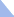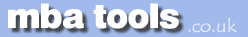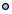MarketingFinanceOperations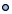EconomicsStatisticsStrategyMeetingsToolboxCommunicationmbatools.co.ukcompiled by

gari jenkins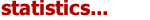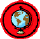Certain types of probability calculations involve dividing the number of outcomes associated with an event by the total number of possible outcomes. For simple problems it is easy to count the outcomes, but in more complex situations manual counting can become laborious or impossible.

Fortunately, there are formulas for determining the number of ways in which members of a set can be arranged. Such arrangements are referred to as permutations or combinations, depending on whether the order in which the members are arranged is a distinguishing factor.

The number of different orders in which members of a group can be arranged for a group of r members taken r at a time is:

(r)(r-1)(r-2)...(1)

This is more easily expressed as simply r!.

When order is a distinguishing factor, a group of n members taken r at a time results in a number of permutations equal to the first r terms of the following multiplication:

(n)(n-1)(n-2)...

This can be expressed as:

nPr = n! / (n - r)!

In combinations, order is not a distinguishing factor:

nC r = nPr / (r!) = n! / (n - r)!r!

For the special case of possible pairs in a group of n members, assuming order in a pair is not important, then:

r = 2

and the number of possible pairs is:

n(n - 1) / 2.

Example: How many two-element subsets of {1,2,3,4} are there that do not contain the pair of elements 2 and 4 ?

Solution: 4! / (2!)(2!) = 6, but the subset {2,4} is not to be counted, so the answer is 5.

Given n items taken r at a time, to find the number of combinations in which x particular items are not present, simply reduce n by x and solve as one would a normal combination problem.

Combinations of Groups

If Group A has x members, Group B has y members, and Group C has z members, there are (x)(y)(z) possible combinations assuming that one member from each of the three groups is used in each combination, and assuming that the order is not a distinguishing factor. In general, if more than one member is taken at a time from each group, the number of combinations is the product of  nCr  (or  nPr  if appropriate) associated with each particular group.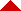Statistics Revision Sheets